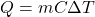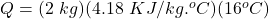## How much energy does it take to raise the temperature of 2kg water from 10 Celsius to 26 Celsius? The specific heat capacity of water is 4.1

Question

How much energy does it take to raise the temperature of 2kg water from 10 Celsius to 26 Celsius? The specific heat capacity of water is 4.18kj/(kg C).

in progress 0
6 months 2021-08-04T20:18:49+00:00 1 Answers 10 views 0

Q = 133.76 KJ

Explanation:

The amount of heat energy required to raise the temperature of the water can be calculated by the following formula:where,

Q = Heat Energy Required = ?

m = mass of water = 2 kg

C = Specific Heat Capacity of Water = 4.18 KJ/kg°C

ΔT = change in temperature = 26°C – 10°C = 16°C

Therefore,Q = 133.76 KJ